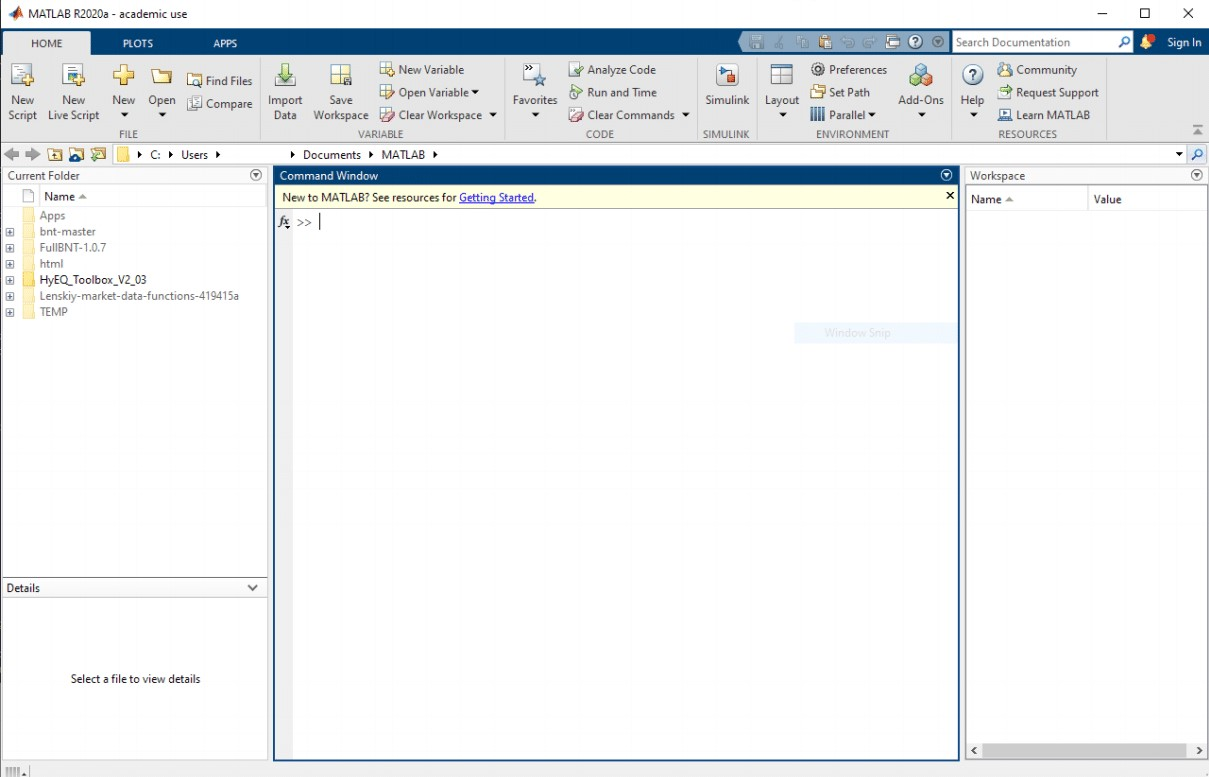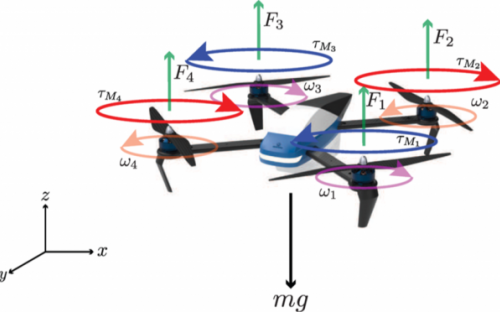# Incorporating STMicroelectronics Drone Kit in UC Santa Cruz’s Robotics Engineering Program

Ricardo G. Sanfelice, University of California Santa Cruz

Project supported by ST Microelectronics

### Introduction

Developing high-quality drone technology is essential for the emerging global market, as drones are able to perform increasingly complex tasks with limited human intervention. Businesses and defense organizations are increasing their investments in drone research to aid in military emergency response, disaster relief, and damage assessment during wildfires, hurricanes, and earthquakes. Utilizing innovative engineering technology to design high-quality, autonomous drones is our goal.

As building drones helps students develop valuable skills in robotics and hardware design, we propose a project to incorporate STMicroelectronics drone kit STEVAL-DRONE01 in our educational program. The goal of the project is to design a curriculum to enrich our Robotics Engineering, specifically, our course ECE8: Robot Automation: Intelligence, through Feedback Control through the use of STMicroelectronics drone kits. To this end, we developed a series of six ECE8 labs, which are detailed below, that to fit teaching modality during the pandemic, can be carried out remotely. This document defines the goals and tasks of the labs from a pedagogical perspective and provides access to the documents to be used during instruction.

### Lab 1 - Basic MATLAB use (Part 1)

This lab introduces students to the world of MATLAB. Tasks include the installation of MATLAB, learning, the ‘help’ command’, basic MATLAB data structures, ‘plot’ command, and ‘for’ loops.• Outcomes: Students learn how to install MATLAB and then start and end a MATLAB session.
• Task 2: Learn to use the ‘help’ command.

• Outcomes: Learn how to use ‘help’ command to see how various functions and commands work.
• Task 3: Learning the basic MATLAB data structures: variables, vectors, matrices, and cells.

• Outcomes: Learn definitions and examples of different MATLAB data types of variables, vectors, matrices, and cells.
• Task 3.1: learn the ‘plot’ command.

• Outcomes: Learn how ‘plot’ command can plot everything from very simple to complex equations.

• Outcomes: Learn how to code a for loop in Matlab.

Full assignment for Lab1 is available here.

### Lab 2 - Basic MATLAB use (Part 2)

This lab introduces students to commonly used ‘control flow’ routines and how to implement them in MATLAB. Full assignment available here.

• Task 1: Create a program that uses ‘if/else’ statements.

• Outcomes: Students use an if/else statement to display one of three messages depending on the value of the variable grade.

• Task 2: Create a program that uses ‘while’ loops.

• Outcomes: Students use a while-loop to divide 1000 by 2 until the result became less than 1, then count and display the number of iterations it took.

### Lab 3 - Introduction to Quadcopters (Part 1)

This lab introduces the simple quadcopter and its components, basic mechanics, and the tools to build simple flight controllers for the one-dimensional and two-dimensional quadcopter systems. Full assignment available here.• Task 1: Identify the key components of a quadcopter and introduce the 1D and 2D models of a quadcopter.

• Outcomes: Students learn the basic components of a quadcopter, including the propeller, motor, Flight Control Unit, and battery.

• Task 2: Use MATLAB to create a program using ‘for/while’ loops and ‘if/else’ statements to simulate a logic controller for height control of a 1D quadcopter model.

• Outcomes: Students learn to simulate one-dimensional quadcopter dynamics by using ‘for/while’ loops and ‘if/else’ statements to control the height of a quadcopter.

• Task 3: Use MATLAB to create a program using ‘for/while’ loops and ‘if/else’ statements to navigate a ‘square’ for a 2D quadcopter model.

• Outcomes: Students simulate two-dimensional quadcopter dynamics by using ‘for/while’ loops and ‘if/else’ statements to navigate a quadcopter in a square.

### Lab 4 - Introduction to Quadcopters (Part 2) and CoppeliaSim

This lab continues the introduction of the dynamics of quadcopters into a three-dimensional space. We also introduce CoppeliaSim, a virtual reality simulation software that allows the simulation of many robotic systems including quadcopters. Full assignment available here.

• Outcomes: Students learn about the vertical, horizontal, and rotational motion of a quadcopter in a 3D coordinate system and model ST Drone as a quadcopter.

• Outcomes: Students learn the basics of accelerometers, gyroscopes, and proximity sensors used in quadcopter applications.
• Task 3: Introduction to CoppeliaSim.

• Outcomes: Students learn CoppeliaSim basics, including how to start sessions, navigate application windows and toolbars, and display scene hierarchy and information text.

• Outcomes: Students learn to identify the variables, parameters, and functions in MATLAB to simulate a quadcopter in CoppeliaSim.
• Task 4: Have students move the target in CopelliaSim and collect the drone position and then create a 3D plot.

• Outcomes: Students use CopelliaSim to move a target and then code a MATLAB script to plot the position of the quadcopter in 3D.

• Outcomes: Students learn how to code a script to move the quadcopter along a square arc through a control algorithm that runs in MATLAB.

• Outcomes: Students learn how to code a script to move the quadcopter along a circular path.

### Lab 5 - Flight Control with Sensors (Part 1)

This lab discusses the proportional-integral-derivative (PID) control as the most common control method. Students are introduced to the PD control, which only uses P and D parameters, for controlling the motion of the quadcopter. Full assignment available here.

• Task 1: Design and tuning of PID controller for ST Drone to reach the target.

• Outcomes: Students learn to control the motion of an ST Drone as a quadcopter to reach a target position.

• Task 2: Redesign PID controllers to track a trajectory generated by a planner.

• Outcomes: Students learn how to design a tracking controller to track a given reference trajectory.

### Lab 6 - Flight Control with Sensors (Part 2)

This lab is a bonus project that exercises the robotics knowledge learned in ECE 8. The project simulates students’ employment as a robotic software and control engineer at a company developing autonomous delivery solutions, and tasks them with developing a demonstration of a delivery scenario. Full assignment available here.